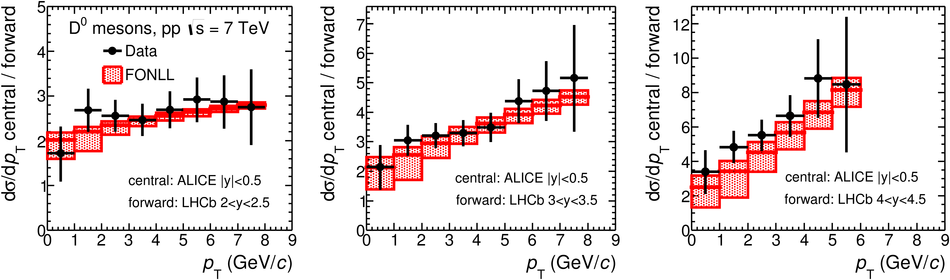Measurement of D-meson production at mid-rapidity in pp collisions at $\mathbf{\sqrt{s}=7}$ TeV

The production cross sections of the prompt charmed mesons ${\rm D^0}$, ${\rm D^+}$, ${\rm D^{*+}}$ and ${\rm D_s^+}$ were measured at mid-rapidity in proton-proton collisions at a centre-of-mass energy $\sqrt{s}=7$ TeV with the ALICE detector at the Large Hadron Collider (LHC). D mesons were reconstructed from their decays ${\rm D}^0 \to {\rm K}^-\pi^+$, ${\rm D}^+\to {\rm K}^-\pi^+\pi^+$, ${\rm D}^{*+} \to {\rm D}^0 \pi^+$, ${\rm D_s^{+}\to \phi\pi^+\to K^-K^+\pi^+}$, and their charge conjugates. With respect to previous measurements in the same rapidity region, the coverage in transverse momentum ($p_{\rm T}$) is extended and the uncertainties are reduced by a factor of about two. The accuracy on the estimated total $\rm c\overline c$ production cross section is likewise improved. The measured $p_{\rm T}$-differential cross sections are compared with the results of three perturbative QCD calculations.

Figures

Figure 1

 Invariant-mass (mass-difference) distributions of $\Dzero$, $\Dplus$, ($\Dstar$) and $\Ds$candidates and charge conjugates in three $\pt$ intervals for a sample of pp collisions at $\sqrt s=7~\tev$with $L_{\rm int}=6.0~{\rm nb}^{-1}$. The curves show the fit functions as described in the text The contribution of reflections for the $\Dzero$ meson is included The values of mean ($\mu$) and width ($\sigma$) of the signal peak arereported together with the signal counts ($S$) in the mass interval ($\mu$- 3$\sigma,\mu + 3\sigma$).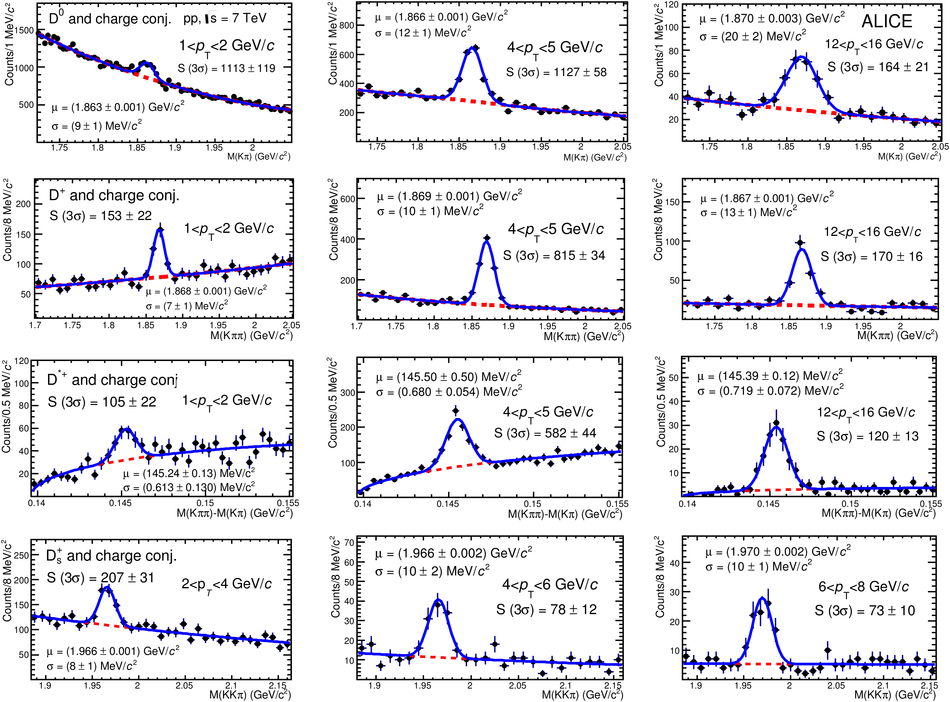Figure 2

 Acceptance $\times$ efficiency for $\Dzero$, $\Dplus$, $\Dstar$ and $\Ds$ mesons, as a function of $\pt$ The efficiencies for prompt (solid lines) and feed-down (dotted lines)D mesons are shown.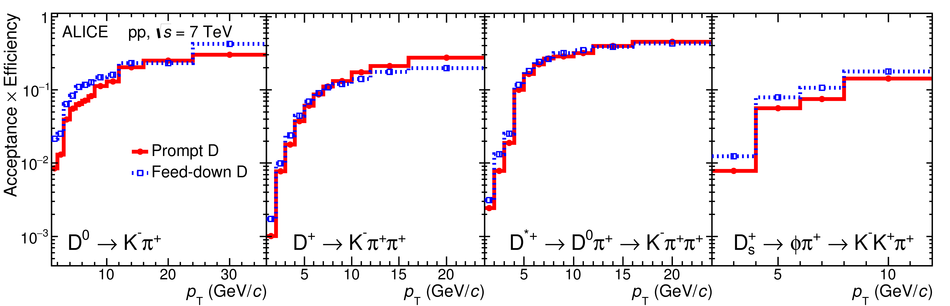Figure 3

 $\pt$-differential inclusive production cross section of prompt $\Dzero$, $\Dplus$, $\Dstar$ and $\Ds$ mesons in pp collisionsat $\sqrts=7~\tev$. Statistical uncertainties (bars) and systematicuncertainties (boxes) are shown. The $\Dstar$ cross section is scaled by afactor of 5 for better visibility.Figure 4

 $\pt$-differential production cross section of prompt $\Dzero$ mesonswith $|y|< 0.5$ in the interval \mbox{$0< \pt< 36~\gev/c$}, in pp collisions at$\sqrt s =7~\tev$ The data point in $0< \pt< 1~\gev/c$ is obtained from the analysis without decayvertex reconstruction described in Ref.~ The cross section is compared to three pQCD calculations:FONLL~ (top-left panel), GM-VFNS~(top-right panel) and a leading order (LO) calculation based on$k_{\rm T}$-factorisation~ (bottom panel) The ratios of the data to the three calculated cross sections are shownin the lower part of each panel. In the data-to-theory ratios the 3.5\%normalisation uncertainty due to the luminosity determination is not includedin the systematic uncertainty on the data pointsFigure 5

 $\pt$-differential production cross section of prompt $\Dplus$ mesonswith $|y|< 0.5$ in the interval \mbox{$1< \pt< 24~\gev/c$}, in pp collisions at$\sqrt s =7~\tev$ The cross section is compared to three pQCD calculations:FONLL~ (top-left panel), GM-VFNS~(top-right panel) and a leading order (LO) calculation based on$k_{\rm T}$-factorisation~ (bottom panel) The ratios of the data to the three calculated cross sections are shownin the lower part of each panel. In the data-to-theory ratios the 3.5\%normalisation uncertainty due to the luminosity determination is not includedin the systematic uncertainty on the data points.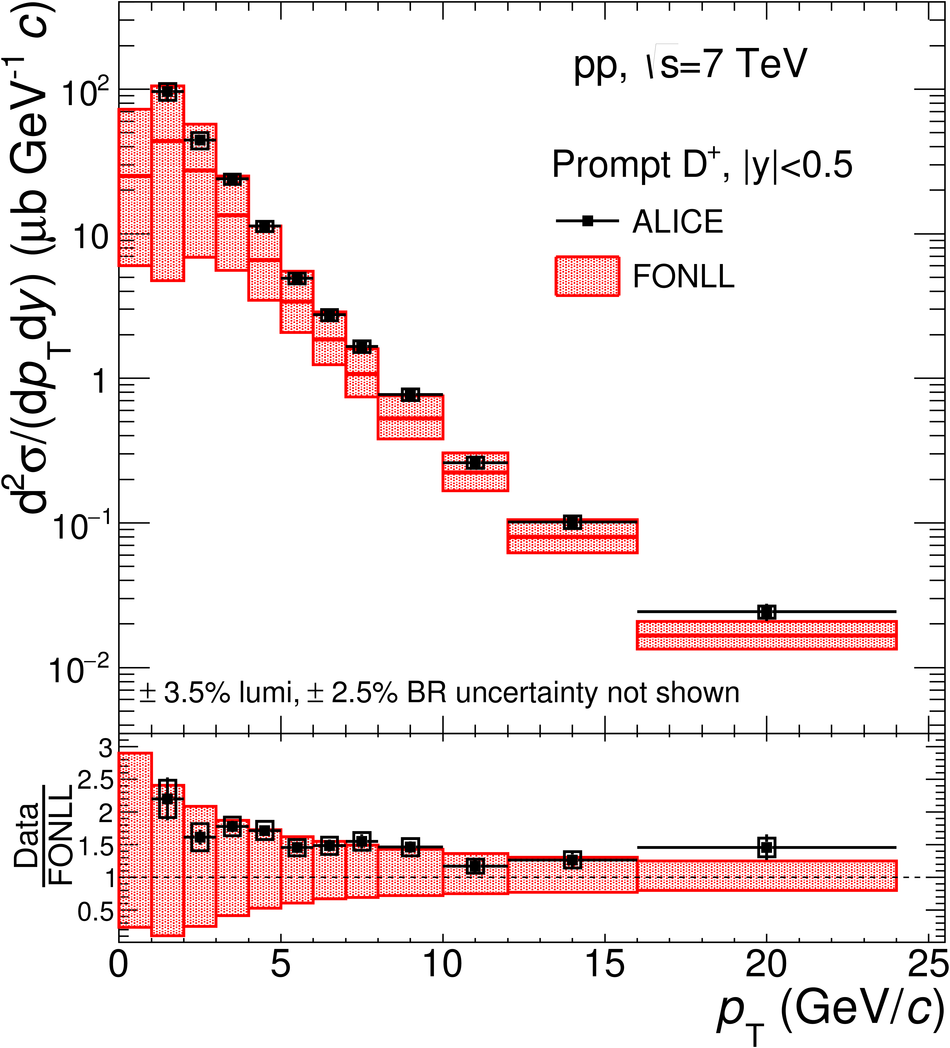Figure 6

 $\pt$-differential production cross section of prompt $\Dstar$ mesonswith $|y|< 0.5$ in the interval \mbox{$1< \pt< 24~\gev/c$}, in pp collisions at$\sqrt s =7~\tev$ The cross section is compared to three pQCD calculations:FONLL~ (top-left panel), GM-VFNS~(top-right panel) and a leading order (LO) calculation based on$k_{\rm T}$-factorisation~ (bottom panel) The ratios of the data to the three calculated cross sections are shownin the lower part of each panel. In the data-to-theory ratios the 3.5\%normalisation uncertainty due to the luminosity determination is not includedin the systematic uncertainty on the data points.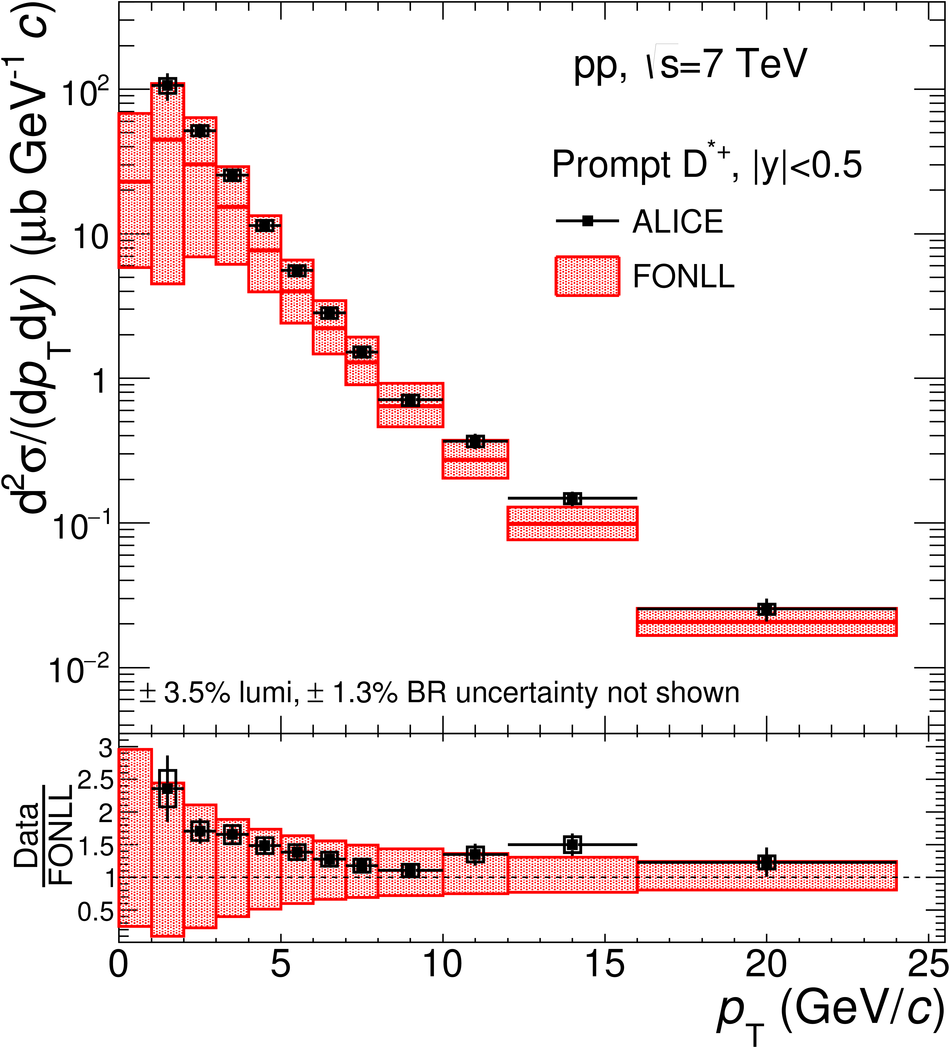Figure 7

 $\pt$-differential production cross section of prompt $\Ds$ mesonswith $|y|< 0.5$ in the interval \mbox{$2< \pt< 12~\gev/c$}, in pp collisions at$\sqrt s =7~\tev$ The cross section is compared to two pQCD calculations:GM-VFNS~ (left panel) and a leading order (LO) calculationbased on $k_{\rm T}$-factorisation~ (right panel) The ratios of the data to the calculated cross sections are shownin the lower part of each panel. In the data-to-theory ratios the 3.5\%normalisation uncertainty due to the luminosity determination is not includedin the systematic uncertainty on the data points.Figure 8

 Ratios of D-meson production cross sections as a function of $\pt$ Predictions from FONLL, GM-VFNS and LO $\kt$-factorisation calculationsare also shown. For the pQCD calculations the line shows the ratio ofthe central values of the theoretical cross sections, while the shaded area isdefined by the ratios computed from the upper and lower limits of thetheoretical uncertainty band.Figure 9

 Ratios of $\Dzero$-meson production cross section as a functionof $\pt$ at mid-rapidity ($|y|< 0.5$) and in threedifferent rapidity intervals at forward rapidity fromLHCb~: $2< y< 2.5$ (left panel),$3< y< 3.5$ (middle panel), $4< y< 4.5$ (right panel) The error bars represent the total (statistical and systematic) uncertaintyon the measurement Predictions from FONLL calculations are compared to the data points.